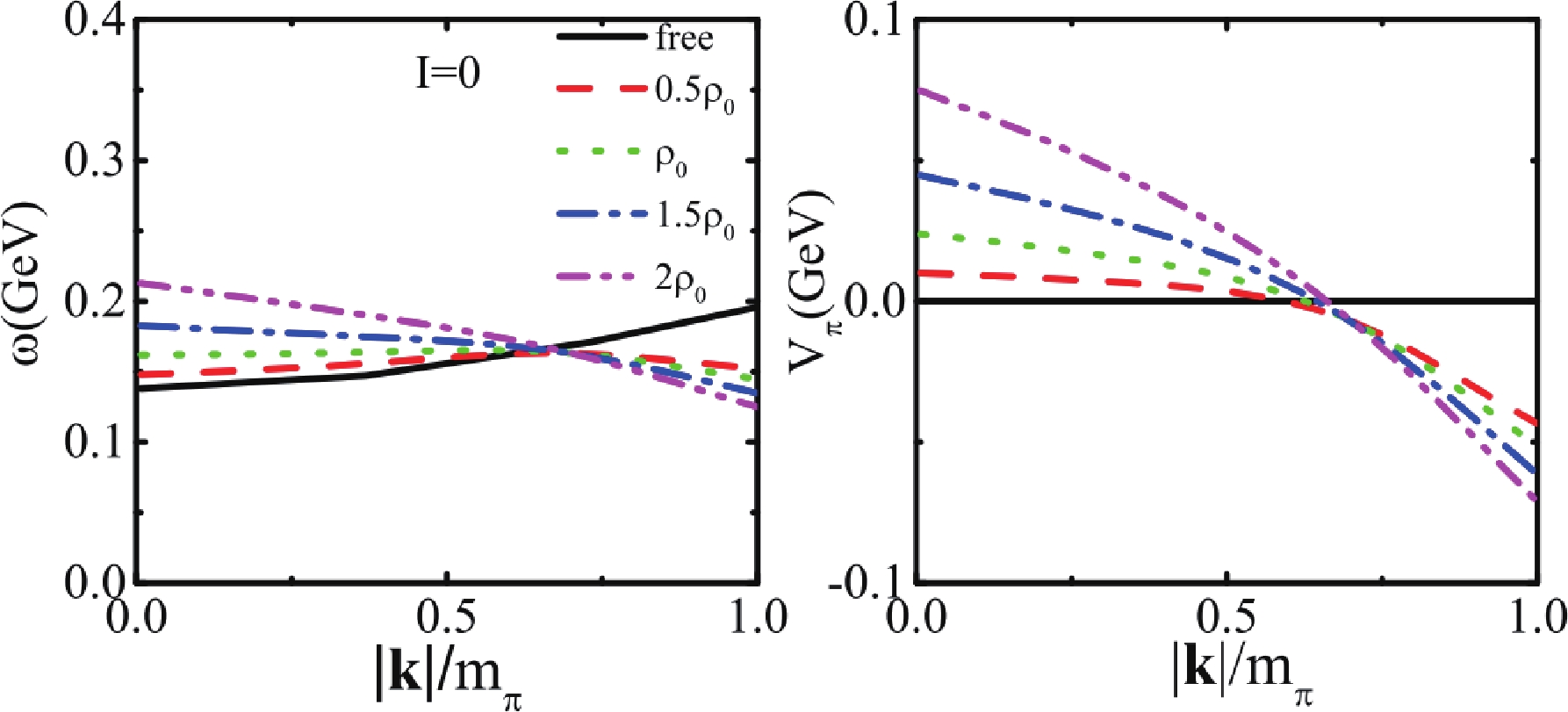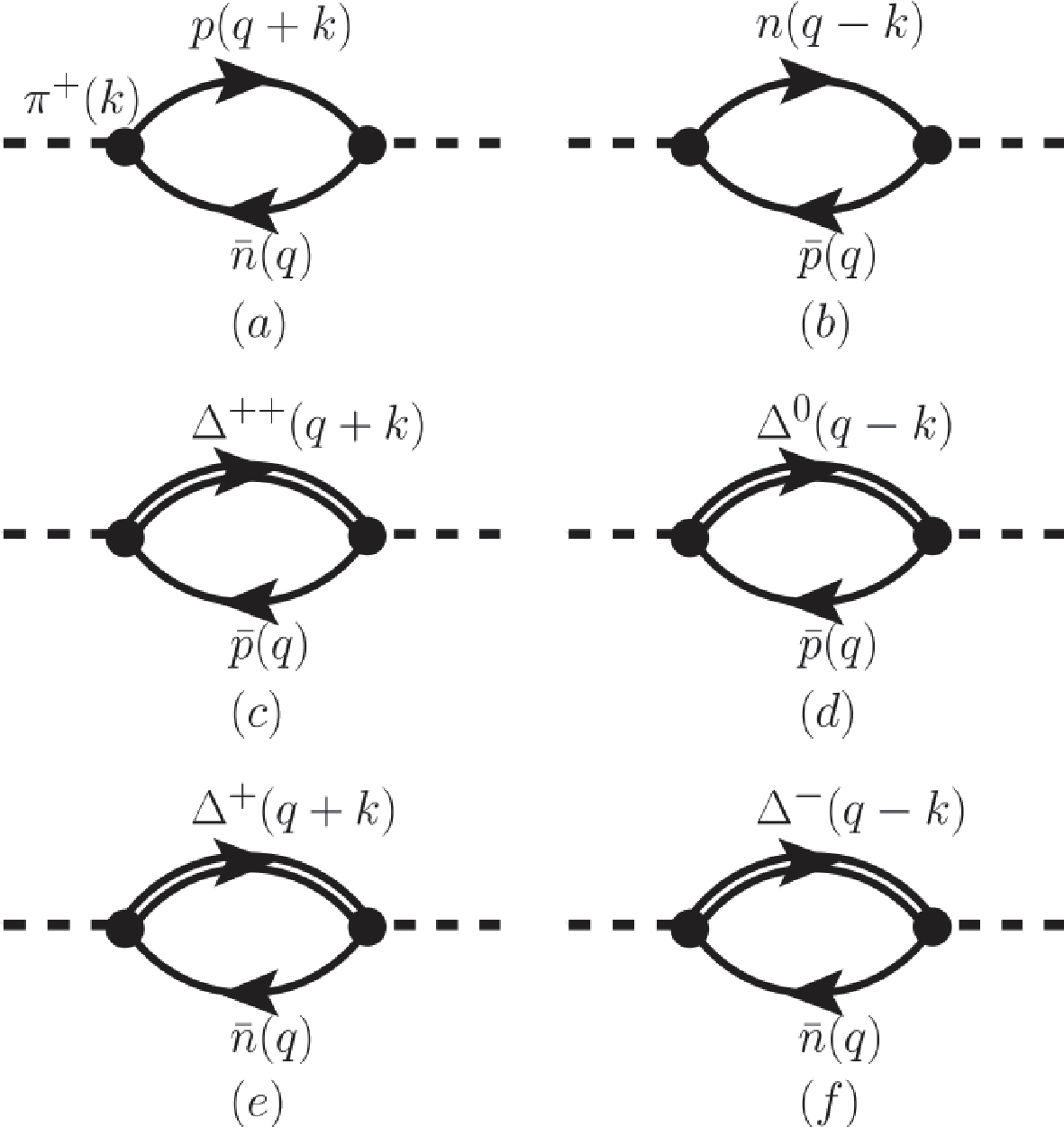# In-medium pion dispersion relation and medium correction of ${{N \pi \leftrightarrow \Delta}}$near the threshold energy of pion production

• Transport models cannot simultaneously explain very recent data on pion multiplicities and pion charged ratios from central collision of Sn+Sn at 0.27 A GeV. This stimulates further investigations on the pion dispersion relation, in-medium $N\pi\to \Delta$cross sections, and $\Delta \to N \pi$decay widths near the threshold energy or at subthreshold energy of pion production in isospin asymmetric nuclear matter. In this study, the pion dispersion relation, in-medium $N\pi\to \Delta$cross section, and $\Delta \to N \pi$decay width near the threshold energy are investigated in isospin asymmetric nuclear matter by using the one-boson-exchange model. With the consideration of the energy conservation effect, the in-medium $N\pi\to\Delta$cross sections are enhanced at $s^{1/2}<1.11$GeV in a nuclear medium. The prediction of pion multiplicity and $\pi^-/\pi^+$ratios near the threshold energy could be modified if this effect is considered in transport model simulations.
••Get Citation
Ying Cui, Ying-Xun Zhang and Zhu-Xia Li. In-medium pion dispersion relation and medium correction of ${{N \pi \leftrightarrow \Delta}}$near the threshold energy of pion production[J]. Chinese Physics C. doi: 10.1088/1674-1137/abe10e
Ying Cui, Ying-Xun Zhang and Zhu-Xia Li. In-medium pion dispersion relation and medium correction of ${{N \pi \leftrightarrow \Delta}}$near the threshold energy of pion production[J]. Chinese Physics C.Milestone
Received: 2020-12-14
Article Metric

Article Views(64)
PDF Downloads(10)
Cited by(0)
Policy on re-use
To reuse of subscription content published by CPC, the users need to request permission from CPC, unless the content was published under an Open Access license which automatically permits that type of reuse.
###### 通讯作者: 陈斌, bchen63@163.com
• 1.

沈阳化工大学材料科学与工程学院 沈阳 110142

Title:
Email:

## In-medium pion dispersion relation and medium correction of ${{N \pi \leftrightarrow \Delta}}$near the threshold energy of pion production

• 1. China Institute of Atomic Energy, Beijing 102413, China
• 2. Guangxi Key Laboratory Breeding Base of Nuclear Physics and Technology, Guangxi Normal University, Guilin 541004, China

Abstract: Transport models cannot simultaneously explain very recent data on pion multiplicities and pion charged ratios from central collision of Sn+Sn at 0.27 A GeV. This stimulates further investigations on the pion dispersion relation, in-medium $N\pi\to \Delta$cross sections, and $\Delta \to N \pi$decay widths near the threshold energy or at subthreshold energy of pion production in isospin asymmetric nuclear matter. In this study, the pion dispersion relation, in-medium $N\pi\to \Delta$cross section, and $\Delta \to N \pi$decay width near the threshold energy are investigated in isospin asymmetric nuclear matter by using the one-boson-exchange model. With the consideration of the energy conservation effect, the in-medium $N\pi\to\Delta$cross sections are enhanced at $s^{1/2}<1.11$GeV in a nuclear medium. The prediction of pion multiplicity and $\pi^-/\pi^+$ratios near the threshold energy could be modified if this effect is considered in transport model simulations.

### HTMLII.   THEORETICAL MODELIII.   RESULTS AND DISCUSSIONSAPPENDIX BAPPENDIX CAPPENDIX D
Reference (55)
PDF查看关注分享

Top

### 目录/DownLoad:  Full-Size Img  PowerPoint